## Mahalanobis Distance

Mahalanobis distance is also called quadratic distance . It measures the separation of two groups of objects. Suppose we have two groups with means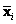and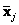, Mahalanobis distance is given by the following

Formula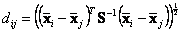The data of the two groups must have the same number of variables (the same number of columns) but not necessarily to have the same number of data (each group may have different number of rows).

In Matlab, the code is as follow:
function d=MahalanobisDistance(A, B)
% Return mahalanobis distance of two data matrices
% A and B (row = object, column = feature)
% @author: Kardi Teknomo
% http://people.revoledu.com/kardi/index.html

[n1, k1]=size(A);
[n2, k2]=size(B);
n=n1+n2;
if(k1~=k2)
disp('number of columns of A and B must be the same')
else
xDiff=mean(A)-mean(B); % mean diff row vector
cA=Covariance(A);
cB=Covariance(B);
pC=n1/n*cA+n2/n*cB; % pooled covariance matrix
d=sqrt(xDiff*inv(pC)*xDiff'); % mahalanobis distance
end

The code above requires computation of Covariance matrix, which code is given below
function C=Covariance(X)
% Return covariance given data matrix X (row = object, column = feature)
% @author: Kardi Teknomo
% http://people.revoledu.com/kardi/index.html

[n,k]=size(X);
Xc=X-repmat(mean(X),n,1); % centered data
C=Xc'*Xc/n; % covariance

## Example

Suppose we have two groups of data, each of group consists of two variables (x, y). The scattered plot of data is shown below.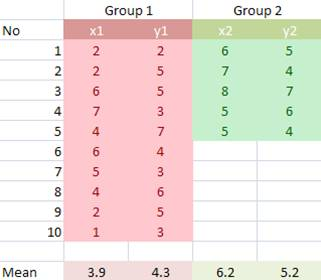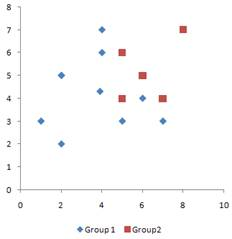First, we center the data on the arithmetic mean of each variable.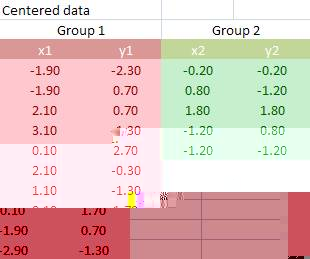Covariance matrix of group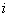is computed using centered data matrix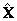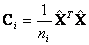It produces covariance matrices for group 1 and 2 as follow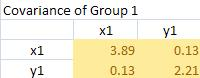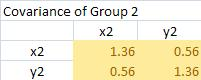The pooled covariance matrix of the two groups is computed as weighted average of the covariance matrices. The weighted average takes this form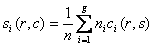.

The pooled covariance is computed using weighted average (10/15)*Covariance group 1 + (5/15)*Covariance group 2 yields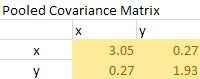The Mahalanobis distance is simply quadratic multiplication of mean difference and inverse of pooled covariance matrix.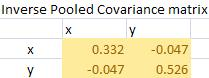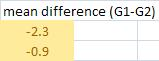To perform the quadratic multiplication, check again the formula of Mahalanobis distance above. When you get mean difference, transpose it, and multiply it by inverse pooled covariance. After that, multiply the result with the mean difference again and you take the square root. The final result of Mahalanobis distance is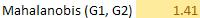Use the interactive program below to compute Mahalanobis distance. If you like this program, please recommend it to your friends.

## How to use the program:

Input are two matrices name matrix A and matrix B that represent features coordinates of two objects. The columns indicate the features, and the rows are the observations. The number of features of the two objects must be equal (i.e. columns of matrix A = columns of matrix B). Each matrix should have at least 2 rows and 1 column.

A matrix is sequence of numbers in a tabular format, inputted using the following format:

• each number in a row is separated by a comma or a space
• each row is separated by semicolon ;

Validate your input before running the program. The initial input values are the example.
Refresh your browser to get back the example.

This program is presented by Kardi Teknomo

Input Matrix A Input Matrix B

Samples of Applications of Mahalanobis Distance